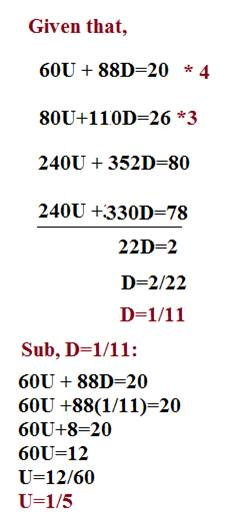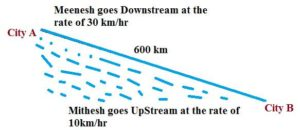# PROBLEMS BASED ON BOATS AND STREAMS-HOW TO SERIES

Hi Bankersdaily Aspirants,

Feeling Good to see you all.How is your Preparation?I think all of them are  Waiting curiously for the exam.Aspirants remember that Practice only Sharpens your mind So Practice well till your exam.Time is So Precious  it will never come again. Don’t Forget Rest is also Equally important so take rest and Study well Brush up what ever you have learnt.Practice Makes man Perfect So Practice well whenever you get time.Today we are going to look problems on Boats & Streams.So Before getting into it let us take a look on Boats & Streams in Learn Series

1)A Man can row 60km Upstream & 88 km downstream in 20 hours.Also,he can row 80km upstream and 110km downstream in 26 hours.Find the rate of the current and the speed of the man in still water?

These type of Problems can be just solved by forming equation,You have to Remember only one thing that,Upstream Speed=1/x and Downstream Speed=1/y

EXPLANATION

Given that,

60U + 88D=20

80U+110D=26Since U=1/X & D=1/Y

X=5.Y=11

Therefore,

Rate in Still Water=(Upstream Speed+DownStream Speed)/2

=(11+5)/2

=16/2

Rate in StillWater=8Km/hr

Rate of Current=(11-5)/2

=6/2

Rate Of Current=3Km/hr

2)City A and B were 600 km Apart.Meenesh live in City A & Mithesh live in City B.The River flows at the rate of 5 km/hr.Meenesh can row a boat at the rate of 25km/hr in still water at the same time Mithesh can row a boat at the rate of 15km/hr in Still water.Find at what time they meet for the first time & Second Time?

EXPLANATION

Given that,

The Cities where 600 Km Apart,

Speed of Meenesh in Still water=25km/hr

Speed of Mithesh in Still water=15km/hr

Speed of Stream=5 km/hr

SPEED OF MEENESH:

DownStream Speed=Speed of Still Water + Speed of Stream =25 + 5 =30 km/hr

UpStream Speed=Speed of Still Water -Speed of Stream=25 -5=20 km/hr

SPEED OF MITHESH:

DownStream Speed=15+5=20km/hr

UpStream Speed=15-5=10km/hrMeenesh & Mithesh will Meet for the First Time:

Here the Distance =600kms

Relative Speed=25+15=40km/hr

Have you Remembered ,what we had written here,

To Cross Each Other when they travel in the Opposite Direction,

=Distance/Relative Speed

=600 km/Relative Speed

When they travel in Opposite Direction their Speeds should be Sum up

When they travel in Same Direction their Speeds should be Subtracted.

=600/40(Because they were travelling in Opposite Direction)

=15 hours

They will meet Each Other after 15 hours of their journey

Their Second Meet:

Meenesh would Reach City B :

Time=Distance/Speed

=600/(25+5)[Downstream Speed=Speed in Still Water+Speed of Stream]

=600/30

=20hours

Meenesh Reach City B after 20 hours

At the same time Mithesh would Reach,

Distance travelled by Mithees=Time*Speed

=20 hours*(15-5)[Because Mithesh is travelling Upstream]

=20*10=200 km

Now,Friends When Meenesh in City B,Mithesh is 200 km ahead of City B.

What is the distance between them,

=200Km,

Speed of Meenesh =25-5=20km/hr(Upstream Speed=Speed in Still Water-Speed of Stream)

Speed of Mithesh=15-5=10km/hr

Relative Speed=200/(20-10)

=200/10

=20hours

They will meet for the Second time at 20+20=40hours